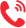更多 >>

学校：
地区：

华东师范大学
• 专业名称
• 所属学院
• 学制
• 学费(元)
• 地区
• 简章详情
• 报名
华中师范大学
• 专业名称
• 所属学院
• 学制
• 学费(元)
• 地区
• 简章详情
• 报名
湖南师范大学
• 专业名称
• 所属学院
• 学制
• 学费(元)
• 地区
• 简章详情
• 报名
石河子大学
• 专业名称
• 所属学院
• 学制
• 学费(元)
• 地区
• 简章详情
• 报名
天津师范大学
• 专业名称
• 所属学院
• 学制
• 学费(元)
• 地区
• 简章详情
• 报名
河北大学
• 专业名称
• 所属学院
• 学制
• 学费(元)
• 地区
• 简章详情
• 报名
山西大学
• 专业名称
• 所属学院
• 学制
• 学费(元)
• 地区
• 简章详情
• 报名
辽宁师范大学
• 专业名称
• 所属学院
• 学制
• 学费(元)
• 地区
• 简章详情
• 报名
沈阳师范大学
• 专业名称
• 所属学院
• 学制
• 学费(元)
• 地区
• 简章详情
• 报名
吉林师范大学
• 专业名称
• 所属学院
• 学制
• 学费(元)
• 地区
• 简章详情
• 报名
齐齐哈尔大学
• 专业名称
• 所属学院
• 学制
• 学费(元)
• 地区
• 简章详情
• 报名
上海师范大学
• 专业名称
• 所属学院
• 学制
• 学费(元)
• 地区
• 简章详情
• 报名
江苏师范大学
• 专业名称
• 所属学院
• 学制
• 学费(元)
• 地区
• 简章详情
• 报名
浙江师范大学
• 专业名称
• 所属学院
• 学制
• 学费(元)
• 地区
• 简章详情
• 报名
杭州师范大学
• 专业名称
• 所属学院
• 学制
• 学费(元)
• 地区
• 简章详情
• 报名
安徽师范大学
• 专业名称
• 所属学院
• 学制
• 学费(元)
• 地区
• 简章详情
• 报名
河南大学
• 专业名称
• 所属学院
• 学制
• 学费(元)
• 地区
• 简章详情
• 报名
广西师范大学
• 专业名称
• 所属学院
• 学制
• 学费(元)
• 地区
• 简章详情
• 报名
重庆师范大学
• 专业名称
• 所属学院
• 学制
• 学费(元)
• 地区
• 简章详情
• 报名
西华师范大学
• 专业名称
• 所属学院
• 学制
• 学费(元)
• 地区
• 简章详情
• 报名
贵州师范大学
• 专业名称
• 所属学院
• 学制
• 学费(元)
• 地区
• 简章详情
• 报名
云南师范大学
• 专业名称
• 所属学院
• 学制
• 学费(元)
• 地区
• 简章详情
• 报名
新疆师范大学
• 专业名称
• 所属学院
• 学制
• 学费(元)
• 地区
• 简章详情
• 报名
广州大学
• 专业名称
• 所属学院
• 学制
• 学费(元)
• 地区
• 简章详情
• 报名
扬州大学
• 专业名称
• 所属学院
• 学制
• 学费(元)
• 地区
• 简章详情
• 报名
江西科技师范大学
• 专业名称
• 所属学院
• 学制
• 学费(元)
• 地区
• 简章详情
• 报名
宁波大学
• 专业名称
• 所属学院
• 学制
• 学费(元)
• 地区
• 简章详情
• 报名
广东技术师范大学
• 专业名称
• 所属学院
• 学制
• 学费(元)
• 地区
• 简章详情
• 报名
四川轻化工大学
• 专业名称
• 所属学院
• 学制
• 学费(元)
• 地区
• 简章详情
• 报名

四川师范大学
• 专业名称
• 所属学院
• 学制
• 学费(元)
• 地区
• 简章详情
• 报名
西北师范大学
• 专业名称
• 所属学院
• 学制
• 学费(元)
• 地区
• 简章详情
• 报名

更多 >>

更多 >>

## 联系方式400-101-7010

报名咨询热线

400-101-7010